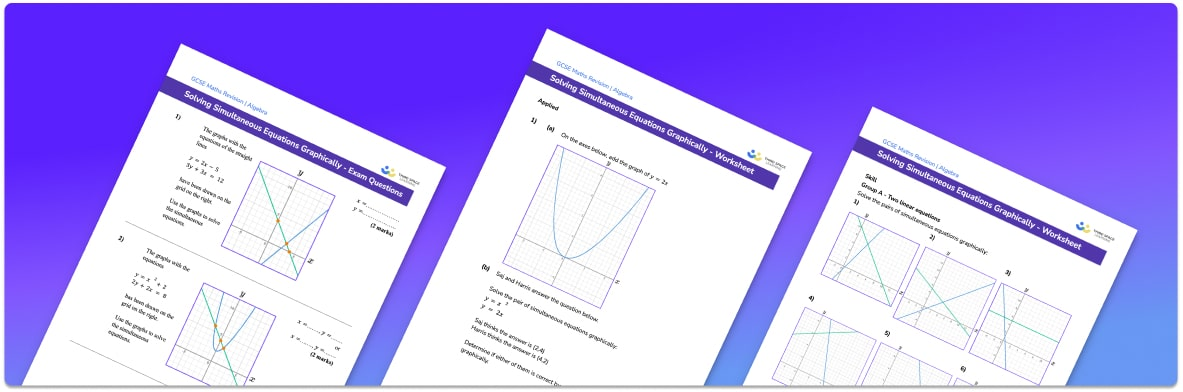# Solving simultaneous equations graphically worksheet• Section 1 of the solving simultaneous equations graphically worksheet contains 20+ skills-based solving simultaneous equations graphically questions, in 3 groups to support differentiation
• Section 2 contains 3 applied solving simultaneous equations graphically questions with a mix of worded problems and deeper problem solving questions
• Section 3 contains 3 foundation and higher level GCSE exam questions on solving simultaneous equations graphically questions
• Answers and a mark scheme for all solving simultaneous equations graphically questions are provided
• Questions follow variation theory with plenty of opportunities for students to work independently at their own level
• All questions created by fully qualified expert secondary maths teachers
• Suitable for GCSE maths revision for AQA, OCR and Edexcel exam boards

• This field is for validation purposes and should be left unchanged.

You can unsubscribe at any time (each email we send will contain an easy way to unsubscribe). To find out more about how we use your data, see our privacy policy.

### Solving simultaneous equations graphically at a glance

Simultaneous equations are pairs of equations with two unknowns. We can solve simultaneous equations to find a set of solutions (one value for each unknown) which work for both equations . For a pair of linear simultaneous equations, there will be one set of solutions. For a pair of equations where one is a linear function and one is a quadratic function, there could be 1 or 2 sets of solutions.

We can solve pairs of simultaneous equations using a graphical method. To do this, we accurately plot the graphs for both equations.  Remember, one way to accurately plot any graph is to create a table of values using the equation for the graph. Once we have plotted the graph for the first equation and the second equation, the coordinates of the point/points of intersection of the graphs will be the solution/solutions to the simultaneous equations

There is no need to match coefficients when solving simultaneous equations graphically (this would be used to solve simultaneous equations by elimination).

Looking forward, students can then progress to additional algebra worksheets, for example a sequences worksheet, simultaneous equations worksheet or straight line graphsFor more teaching and learning support on Algebra our GCSE maths lessons provide step by step support for all GCSE maths concepts.

## Do you have KS4 students who need more focused attention to succeed at GCSE?There will be students in your class who require individual attention to help them succeed in their maths GCSEs. In a class of 30, it’s not always easy to provide.

Help your students feel confident with exam-style questions and the strategies they’ll need to answer them correctly with our dedicated GCSE maths revision programme.

Lessons are selected to provide support where each student needs it most, and specially-trained GCSE maths tutors adapt the pitch and pace of each lesson. This ensures a personalised revision programme that raises grades and boosts confidence.

Find out more